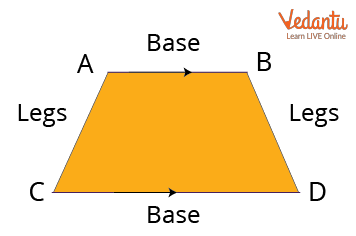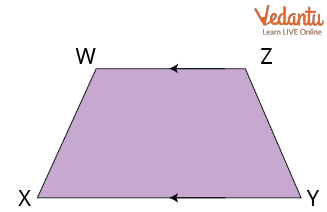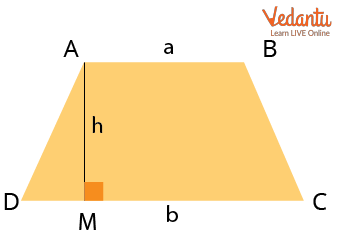Courses
Courses for Kids
Free study material
Free LIVE classes
More

# Perimeter of TrapeziumLIVE
Join Vedantu’s FREE Mastercalss

## Introduction of Trapezium and Its Properties

Have you ever seen a trapezium shape in your daily life?Is it Trapezium?

Can you mention a few examples of trapezium or trapezoid? Have a look at your surroundings and decide on the shape of a trapezium. Would you be able to find out any? A little bit confused between trapezium or trapezoid? No worries, you’ll learn the same here. Firstly, trapezium and trapezoid are the same in American and Canadian English, a trapezoid is a quadrilateral with at least one pair of parallel sides. A trapezoid is known as a trapezium in British and other forms of English.

## Trapezium

A trapezium is a quadrilateral with one pair of parallel opposite sides, as defined by Euclidean geometry. Trapezium comes from the Greek word "trapeza," which means "table." So, the Trapezium is a four-sided shape with only two parallel sides.Trapezium

A trapezium is a two-dimensional quadrilateral with a pair of parallel opposite sides (because of its four straight lines). The base and the non-parallel sides of the trapezium are referred to as the base and legs of the trapezium, respectively.

It has four sides and four corners and is a closed plane shape. The following is a diagram of a trapezium shape:Trapezium WXYZ

In the above figure, XY and WZ are the bases while XW and YZ are the legs of the trapezium.

## Properties of a Trapezium

Each quadrilateral has its unique set of characteristics that distinguish it from the other. These attributes provide extra information about a shape's geometrical construction. The following are the properties of trapezium:

• It's a two-dimensional shape.

• A trapezium's bases are parallel to one another.

• Both diagonals have the same length.

• A trapezium's diagonals are always intersected by each other.

• The total of the adjacent internal angles is 180°.

• In a trapezium, the sum of all internal angles is always 360°.

## Trapezium Formula

Consider a trapezium with the lengths of parallel sides a and b units and the altitude "h."Trapezium with the Lengths of Parallel Sides and the Altitude

Calculating the average of bases and multiplying the result by the altitude yields the trapezium area. As a result, trapezium area $= \left(\dfrac{AB + DC}{2}\right) AM = \left(\dfrac{a+b}{2}\right) h$, where AB, CD represent the bases and AM represents the altitude.

The perimeter of a trapezium is calculated by adding all of its sides together (because the perimeter is the sum of boundaries). As a result, the perimeter of a trapezium ABCD = AB + BC + CD + AD.

## Solved Examples

1. If a trapezium is given with its three sides whose measures are 10cm, 12cm, and 7cm. Find the length of the fourth side of the trapezium if the perimeter is 40 cm.

Ans: Perimeter of trapezium = Sum of all its sides (all four sides) = 40 cm

Let the fourth side be ‘a’ cm.

Thus, applying the formula:

Perimeter is 40 = 10 + 12 + 7 + a

a = 40 – (10 + 12 + 7)

a = 40 – 29

a = 11 cm

Therefore, the length of the fourth side is 11 cm.

2. Seeta bought a trapezium-shaped table whose bases and legs are 25 cm,20 cm, 10 cm, and 12 cm respectively. She wants to know the perimeter of the table. Can you help her to find it?

Ans: Perimeter of trapezium = Sum of all its sides

So, applying the formula:

Perimeter of trapezium = 25 + 20 + 10 + 12

Perimeter of trapezium = 67 cm

Hence, the perimeter of the table is 67 cm.

Q1. The parallel sides of a trapezium are 25 cm and 11 cm, while its nonparallel sides are 15 cm and 13 cm. Find the perimeter of the trapezium.

Ans: 64 cm

Q2. What is the perimeter of the trapezoid in which the sum of lengths of non-parallel sides is 12 units, and the sum of the parallel sides is 8 units?

Ans: 20 units

## Conclusion

Trapezium comes from the Greek word "trapeze," which means "table." So, the Trapezium is a four-sided shape with only two parallel sides. The perimeter of a trapezium is calculated by adding all of its sides together (because the perimeter is the sum of boundaries). As a result, the perimeter of a trapezium ABCD = AB + BC + CD + AD.

Last updated date: 24th Sep 2023
Total views: 121.8k
Views today: 2.21k

## FAQs on Perimeter of Trapezium

1. What are the properties of a trapezium?

The properties of a trapezium are given below:

• It's a two-dimensional shape.

• A trapezium's bases are parallel to one another.

• Both diagonals have the same length as they are always parallel.

• A trapezium's diagonals are always intersected by each other.

• The total of the adjacent internal angles is 180°.

• In a trapezium, the sum of all internal angles is always 360°.

2. What is a trapezium? Who invented it and when?

A trapezium shape is a two-dimensional quadrilateral with a pair of non-adjacent parallel sides and a pair of non-parallel sides. The trapezium was invented by J.P. Smith in 1813 when he came up with the trapezium (a quadrilateral with no two sides parallel). The trapezius is named for its trapezoidal shape. It is flat and broad, covering the upper back and part of the neck and shoulders, but it's actually part of the thorax. From this, it got the name trapezium.